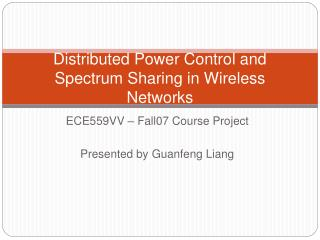DownloadDownload PresentationDistributed Power Control and Spectrum Sharing in Wireless Networks

# Distributed Power Control and Spectrum Sharing in Wireless Networks

Télécharger la présentation## Distributed Power Control and Spectrum Sharing in Wireless Networks

- - - - - - - - - - - - - - - - - - - - - - - - - - - E N D - - - - - - - - - - - - - - - - - - - - - - - - - - -
##### Presentation Transcript

1. Distributed Power Control and Spectrum Sharing in Wireless Networks ECE559VV – Fall07 Course Project Presented by Guanfeng Liang

2. Outline • Background • Power control • Spectrum sharing • Conclusion

3. Background • Interference is the key factor that limits the performance of wireless networks • To handle interference, can optimize by means of • Frequency allocation: • Power control: • Or, jointly - spectrum sharing: f f f

4. Power Control • N users, M base stations, single channel, uplink • Pj - transmit power of user j • hkj - gain from user j to BS k • zk – variance of independent noise at BS k

5. General Interference Constraints • Fixed Assignment: BS aj is assigned to user j • Minimum Power Assignment: each user is assigned to the BS that maximizes its SIR • Limited Diversity: BS’s in Kj are assigned to user j

6. Standard Interference function • Definition: Interference function I(p)is standard if for all p≥0, the following properties are satisfied. • Positivity - I(p) ≥0 • Monotonicity - If p ≥ p’, then I(p)≥I(p’). • Scalability – For all a>1, aI(p)>I(ap). • IFA, IMPA, ILD are standard. • For standard interference functions, minimized total power can be achieved by updating p(t+1)=I(p(t)) in a distributed fashion, asynchronously. (Yates’95)

7. Spectrum Sharing Power is uniformly allocated across bandwidth W Transmission rate is not considered What should we do if power is allowed to be allocated unevenly? Can “rate” optimality be achieved in a distributed manner?

8. Settings • M fixed 1-to-1 user-BS assignments • Noise profile at each BS: Ni(f) • Random Gaussian codebooks – interference looks like Gaussian noise

9. Rate Region • Rate Region • Pareto Optimal Point

10. Optimization Problem • Global utility optimization maximization • U(R1,…,RM) reflects the fairness issue • Sum rate: Usum (R1,…,RM) = R1+…+RM • Proportional fairness: UPF (R1,…,RM) = log(R1)+…+log(RM) • In general, U is component-wise monotonically increasing => optimal allocation must occur on the boundary R*

11. Examples

12. Infinite Dimension • Theorem 1: Any point in the achievable rate region R can be obtained with M power allocations that are piecewise constant in the intervals [0,w1), [w1,w2),…,[w2M-1,W], for some choice of {wi}i=1.2M-1. • Theorem 2:Let (R1,…,RM) be a Pareto efficient rate vector achieved with power allocations {pi(f)}i=1,…,M. If hi,jhj,i>hi,ihj,j then pi(f)pj(f)=0 for all f [0,W].

13. Non-Cooperative Scenarios • Non-convex capacity expression -> rate region not easy to compute • Another approach: view the interference channel as a non-cooperative game among the competing users-> competitive optimal • Assumptions: • Selfish users • user i tries to maximize Ui(Ri) -> maximize Ri

14. Gaussian Interference Game(GIG) • Each user tries to maximize its own rate, assuming other users’ power allocation are known. • Well-known Water-filling power allocation

15. Iterative Water-filling (Yu’02)

16. Equilibrium • Theorem 3:Under a mild condition, the GIG has a competitive equilibrium. The equilibrium is unique, and it can be reached by iterative water-filling. • Nash Equilibrium

17. Is the Equilibrium Optimal? • NO! • Example: • h1,1=h2,2=1, h1,2=h2,1=1/4, W=1, N1=N2=1, P1=P2=P • Water-filling -> flat power allocation: • Orthogonal power allocation

18. Repeated Game • Utility of user i : • Decision made based on complete history • Advantage: much richer set of N.E., hence have more flexibility in obtaining a fair and efficient resource allocation

19. Equilibriums of a Repeated Game • Fact: frequency-flat power allocations is a N.E. of the repeated game with AWGN. • Theorem 4:The rate RiFS achieved by frequency-flat power spread is the reservation utility of player i in the GIG. • Result: If the desired operating point (R1,…,RM) is component-wise greater than (R1FS,…,RMFS), there is no performance loss due to lack of cooperation. (Tse’07)

20. Results

21. Summary • Performance optimization of wireless networks • 1-D: power = power control • Distributed power control with constant power allocation • 2-D: power + frequency = spectrum sharing • One shot GIG – iterative water-filling • Repeated game • 3-D: power + frequency + time • Cognitive radio

22. Thank you and Questions?